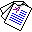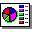# Inductive Equivalence of Logic Programs

## Chiaki Sakama and Katsumi Inoue

Proceedings of the 15th International Conference on Inductive Logic Programming (ILP'05), Lecture Notes in Artificial Intelligence 3625, pages 312-329, Springer-Verlag, 2005.

## Abstract

This paper studies equivalence issues in inductive logic programming. A background theory B1 is inductively equivalent to another background theory B2 if B1 and B2 induce the same hypotheses for any given set of examples. Inductive equivalence is useful to compare inductive capabilities among agents having different background theories. Moreover, it provides conditions for optimizing background theories through appropriate program transformations. In this paper, we consider three different classes of background theories: clausal theories, Horn logic programs, and nonmonotonic extended logic programs. We show that logical equivalence is the necessary and sufficient condition for inductive equivalence in clausal theories and Horn logic programs. In nonmonotonic extended logic programs, on the other hand, strong equivalence is necessary and sufficient for inductive equivalence in general. Interestingly, however, we observe that several existing induction algorithms require weaker conditions of equivalence under restricted problem settings. We also discuss connection to equivalence in abductive logic and conclude that the notion of strong equivalence is useful to characterize equivalence of non-deductive reasoning.Full Paper (pdf 242K)Slide (pdf 171K) , © Springer-Verlag